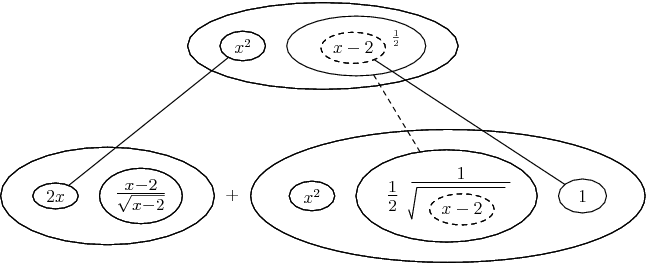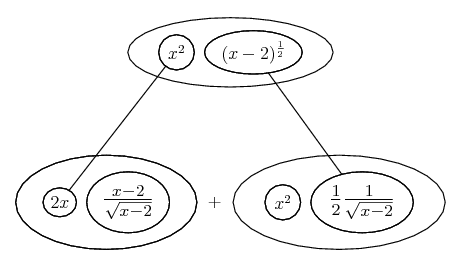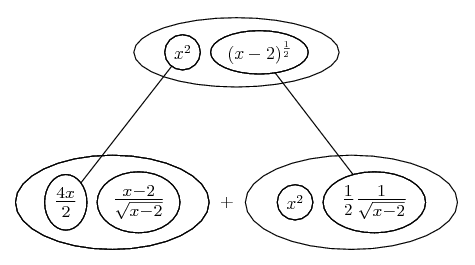# Thread: Derivatives - product rule + chain rule

1. ## Derivatives - product rule + chain rule

I'm having trouble getting to the derivative of $\displaystyle y = x^2 sqrt(x-2)$. Could someone kindly provide a step-by-step solution to the problem? The answer is $\displaystyle x(5x - 8) / 2 sqrt(x-2)$

2. Are you sure? Here's a pic - I'll edit details and quite possibly corrections...Actually, the chain rule here is kind of trivial, so we could just use the product rule alone...... where...... is the product rule. Straight continuous lines differentiate downwards (integrate up) with respect to x, and then, because of the product rule, the whole of the bottom is the derivative of the whole of the top.

Notice I've tweaked the second balloon along the bottom, just for a common denominator.

OK - where does that get us in view of your proposed answer? Have you checked question and answer? Maybe its me...

__________________________________________

Don't integrate - balloontegrate!

http://www.ballooncalculus.org/examples/gallery.html

http://www.ballooncalculus.org/asy/doc.html

3. What's the problem?

If the provided answer caused some confusion, it should be: $\displaystyle x(5x - 8) / (2 sqrt(x-2))$ to be more specific.

4. Sorry, should have thought to do an additional tweak for the same reason, then I wouldn't have been confused...So, yes, there we are. Maybe your mental blip was related to mine. Anyway, hope you like the balloons for tracing through the product rule process - if not, use the formula, of course.

5.Originally Posted by tom@ballooncalculusSorry, should have thought to do an additional tweak for the same reason, then I wouldn't have been confused...So, yes, there we are. Maybe your mental blip was related to mine. Anyway, hope you like the balloons for tracing through the product rule process - if not, use the formula, of course.
I'm sorry I find the balloons kind of confusing. How did you get 4x/2 from x^2?

6. That was the additional tweak for the sake of the common denominator. (2x because it's the derivative of x^2, then I just doubled the top and bottom.)

Don't be sorry! - find an approach that works for you...

Cheers

7. Ah I finally understand - thank you so much!

#### Search Tags

chain, derivatives, product, rule